## Differentiable reasoning over text

by
June 3, 2020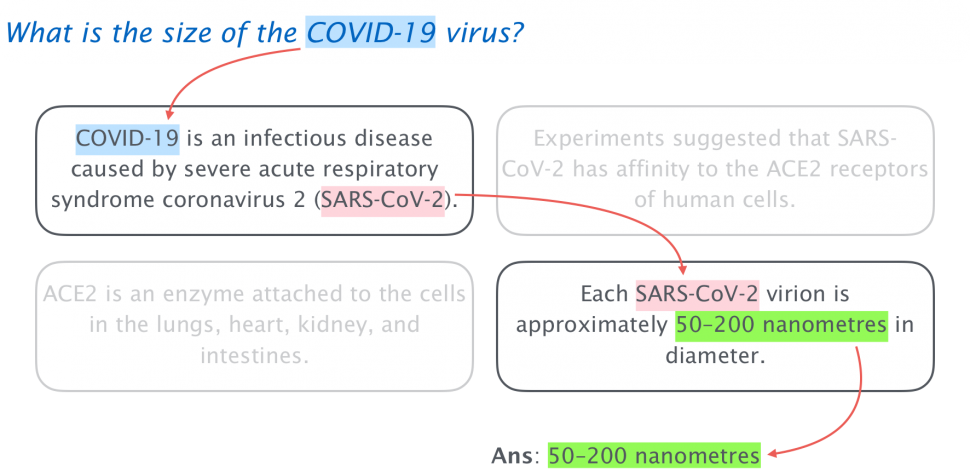By Bhuwan Dhingra

We all rely on search engines to navigate the massive amount of online information published every day. Modern search engines not only retrieve a list of pages relevant to a query but often try to directly answer our questions by analyzing the content of those pages. One area they currently struggle at, however, is multi-hop Question Answering that requires reasoning with information taken from multiple documents to arrive at the answer. For example, suppose that we want to find out What is the size of the COVID-19 virus?. Systems that try to answer this question first need to identify the virus responsible for COVID-19 (SARS-CoV-2) and then look for the size of that virus (50-200 nm). These two pieces of information may be mentioned in different documents, making the task challenging for conventional approaches. In this blog post, based on our recent ICLR paper, we describe a fast, end-to-end trainable model for answering such multi-hop questions.

Most of the existing work on question answering (QA) falls in two broad categories. Retrieve + Read systems take the documents returned by standard search engines and run a deep neural network over them to find a span of text that answers the question. However, for multi-hop questions all the relevant documents may not be retrieved by the search engine in the first place, limiting the abilities of these systems. In the example above, the document that talks about the size of SARS-CoV-2 does not share any words with the question and might be missed out.

Another line of work has looked at Knowledge-Based QA, where documents are first converted into a knowledge base (KB) by running information extraction pipelines. A KB is a graphical data structure whose nodes and edges correspond to real-world entities and the relationships between those entities, respectively. Given a sufficiently rich KB, multi-hop questions can be efficiently answered by traversing its edges. However, constructing KBs is an expensive, error-prone and time-consuming process: new information (such as about COVID) first appears on the web in textual forms and adding it to KBs involves a delay. Furthermore, not all information can be expressed using the predefined set of relations allowed by a KB, and hence KBs are usually incomplete.

Motivated by the aforementioned limitations, we explore whether we can directly treat a text corpus as a KB for answering multi-hop questions. At a high level, to achieve this goal we first convert the corpus into a graph-structure similar to a KB while keeping the documents in their original form. We then define a fast, differentiable operation for traversing the edges in the graph, which we call relation following. Given a question, we finally decompose it into a sequence of relations that informs the graph traversal and leads to the answer. Our design ensures that the traversal operation is differentiable, thereby allowing end-to-end training of the entire system. We also introduce an efficient implementation of the traversal operation that scales to the entire Wikipedia in milliseconds!

### From Text to Graph

To answer a multi-hop question, we aim to traverse a sequence of relevant facts through the text corpus. In order to do this, we first need some way to identify the connections between the different passages in the corpus. One way to do this is by identifying a list of important terms and concepts in our corpus, also known as entities, and connecting their different mentions to each other. This construction is known as entity linking and can be represented as a graph like the one shown in Figure 1 below.Figure 1: An entity linked corpus can be viewed as a bipartite graph with entities on the right and their mentions in texts on the left. Note that an entity can be linked to multiple mentions.

The difference between this graph and a KB is that we leave the documents in their original form instead of encoding them in a fixed set of relationships. Hence, our graph structure is cheaper to construct and remains as expressive as the original text while incorporating the structure inherent in a KB. In the next section, we introduce a procedure that employs this graph structure to answer questions by moving from entities to mentions and back multiple times.

### Relation Following

The key idea behind our approach is that we can formulate QA as a path-finding problem in the graph above. The path originates from a set of question-specific entities and then follows a carefully constructed sequence of relations to arrive at the answer. The number of relations in the path depends on the number of hops in the question: for a single-hop question we need to travel between the entities and the text once, and multi-hop questions may require multiple traversals.

To introduce the traversal operation, suppose we are at a set of entities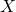and we need to follow the relation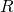in the graph. The traversal then proceeds in three steps:

1. Jump from entities to mentions: Given the starting set of entities, we identify all the mentions in the text passages that co-occur with at least one member of.
2. Filter mentions based on: Among the co-occurring mentions, we identify the ones that satisfy the relationwith one of the starting entities. We do so by learning a scoring function that compares the contextual embedding of the mention to an embedding constructed from the relation.
3. Jump from mentions back to entities: For each entity that is connected to some of the filtered mentions, we compute its weight by aggregating scores from all the corresponding mentions. The result is a weighted set of entities, where the weights denote the confidence that an entity satisfies the relationwith a member of.

Formally, we introduce a relation following operation defined over sets of entities:Starting at a set of entities, we “follow” the relationto arrive at an output set of entities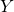, such that each member ofis related to at least one member ofthrough the relation. Importantly, we do not restrictto be from a predefined set and allow relations to be described in natural language.

Cohen et al (2019) used an implementation of this operation to answer multi-hop questions over a KB. In our paper, we introduce an implementation over an entity linked text corpus. Our implementation has some important properties making it suitable for multi-hop QA:

• Fast: We only rely on sparse matrix multiplications and a maximum inner product search (MIPS). Thus, the overall complexity depends only on the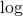of the number of mentions in the corpus.
• Compositional: Both the input and output of the operation are weighted sets of entities. We can, therefore, chain the operations into complex templates for multi-hop QA, e.g.,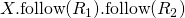.
• Differentiable: The weights of the output entities are differentiable w.r.t the weights of the input entities and the embedding of the relation. This allows us to backpropagate gradients and train our system end-to-end.

Let us now give more details on our implementation of relation following. We represent our starting set of entitiesas a sparse vector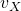, whose size equals the number of entities in the corpus and whose non-zero elements correspond to the elements of. We also encode the relationinto a continuous embedding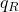. With this notation, our implementation can be written compactly in a single equation: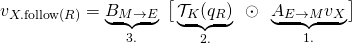Each term in this equation corresponds to one of the steps in the procedure above, as indicated by the numbers below the terms. Jumping from entities to mentions (step 1) can be implemented as a product of a sparse matrix and a sparse vector. Let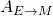denote a sparse matrix that maps entities to their co-occurring mentions. As illustrated in Figure 2 below,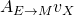then is the set of mentions that co-occur with.

For step 2, we need to filter mentions based on their relevance to the relation. To do this efficiently, we preprocess the corpus into an offline index of embeddings for each detected mention. These embeddings are obtained by passing the entire passage through a BERT model, which is fine-tuned on simple questions, and extracting the representations corresponding to the tokens in the mention. After indexing all the mentions, we end up with an embedding table of size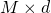, where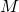is the total number of mentions and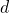is the size of each embedding.

Given the embeddingof the relation, we then run a fast MIPS algorithm to find the top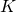mentions from the index that match this relation. The result can be expressed as another sparse vector, which we denote as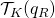, whose size is again the number of mentions in the corpus, with exactlynon-zero elements corresponding to the nearest neighbors of, as illustrated in Figure 3 below.

We take an element-wise product (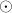) between the sparse vectors obtained in steps 1 and 2, intersecting the two sets of mentions obtained in each step. Finally, we jump back from mentions to entities (step 3) using another sparse matrix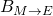that maps each mention to the entity that it refers to. The result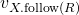is another sparse vector representing the weighted set of entities. Here the weights depend on the inner product score between the mentions of that entity and the relation, and represent the confidence that an entity satisfies the relationwith a member of.

### End-to-End Multi-Hop QA

We have introduced a fast, compositional and differentiable implementation of the traversal operation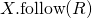. To use it for answering a multi-hop question, we extract a starting set of entitiesand a sequence of relation embeddings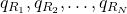from the question. We then apply the relation following operation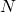times to derive the answer. Since the operation is differentiable, we can train the components that derive the entities and relation embeddings using a loss computed over the final answer! Concretely, given a question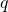, we derive entities and relations as: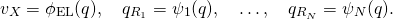Here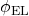can be any entity linking system, and we employ Transformer networks to generate relation embeddings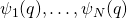, training the parameters of these networks using cross-entropy loss computed on the final answer. Importantly, we do not need any supervision for the starting entities or the sequence of relations.

### Demo!

Below we show a real-time demo of our end-to-end system running on a single 16-core CPU. The underlying corpus consists of all introductory passages on Wikipedia (>5M). Given a question, we run our system for a maximum of 3 hops to identify the top 5 relevant passages in Wikipedia. Then we run another BERT model to extract a span of text as the answer and also identify the sentences that support it (highlighted in yellow). All models are trained on the HotpotQA dataset.

We refer the reader to our paper for a detailed quantitative comparison to other QA systems on the multi-hop benchmarks MetaQA and HotpotQA. In summary, on MetaQA our model outperforms the previous best by 6% and 9% on 2-hop and 3-hop questions, respectively, while being up to 10x faster. On HotpotQA, our method trades off some accuracy (F1 score 43 vs 61) to deliver a 100x improvement in terms of speed, making the inference seamless for the end user. The gain of speed comes from the fact that our method allows indexing the corpus offline, saving inference time as we only call the costly BERT once, in contrast to 50-500 BERT calls required by other systems.

### What’s Next?

Our work naturally entails a couple of interesting directions for future research:

1. Richer templates: We focused solely on templates that chain a sequence of relation following operations. In principle, our system can be easily extended to allow templates that take a union or intersection of sets, by adding or multiplying the sparse vectors representing them. But real KBs allow a much richer class of operations, such as negation, filtering, argmax and counting. There has been some work on implementing differentiable versions of these over KBs and one promising direction is to implement similar operations over text.
2. Richer representations: The speed of our system comes, in part, from populating the offline index with embeddings of the mentions that are independent of the queries. In contrast, query-dependent embeddings typically show higher performance. Hence, another important direction is to bridge the gap in performance caused by question-independent encodings of mentions we rely on in this work.

For more details and a link to the code, please check our paper here. A video of the conference talk is available here.

DISCLAIMER: All opinions expressed in this post are those of the author and do not represent the views of CMU.

This article was initially published on the ML@CMU blog and appears here with the authors’ permission.